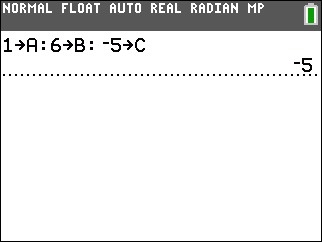# Knowledge Base

## Solution 34620: Finding the Discriminant of a Quadratic Equation on the TI-83 Plus and TI-84 Plus Family of Graphing Calculators.

### How can I find the discriminant of a quadratic equation on the TI-83 Plus and TI-84 Plus family of graphing calculators?

The TI-83 Plus and TI-84 Plus family of graphing calculators do not have the built-in function to find the discriminant. However, the instructions below show how to compute the discriminant by using variables:

The discriminant is a number that can be calculated from any quadratic equation. A quadratic equation is an equation that can be written as ax2 + bx + c = 0

The discriminant in a quadratic equation is found using the formula below and the discriminant provides critical information regarding the nature of the roots/solutions of any quadratic equation:

discriminant = b-4ac

Critical information on the solutions:

• When b2-4ac = 0 then there are 2 equal roots.
• When b2-4ac > 0 then there are 2 different roots.
• When b2-4ac < 0 then there are no roots at all.

Example: Find the discriminant of the equation x2+6x-5=0

1) Press  [sto→] [alpha] [math] [alpha] [.]  [sto→] [alpha] [apps] [alpha] [.] [ (-) ]  [sto→] [alpha] [prgm] [enter]2) Press [alpha] [apps] [x2] [-]  [*] [alpha] [math] [*] [alpha] [prgm] [enter] to display the discriminant. For this equation, the discriminant is 56.Please see the TI-83 Plus and TI-84 Plus Family guidebooks for additional information.

Last updated: 7/14/2023